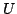Statistica Sinica 16(2006), 1409-1422

EXACT CONVERGENCE RATE AND LEADING TERM IN

THE CENTRAL LIMIT THEOREM FOR-STATISTICS

Qiying Wang and Neville C Weber

The University of Sydney

Abstract: The leading term in the normal approximation to the distribution of-statistics of degree 2 is derived. This result is applied to establish the exact rate of convergence in the Central Limit Theorem for-statistics and to obtain the one-term Edgeworth expansion for the distribution function. Analogous results for more general-type statistics are also considered.

Key words and phrases: Berry-Esséen theorem, characterisation of rate of convergence, Edgeworth expansion, optimal moments, nonlattice condition, U-statistics, L-statistics.